### Home > CALC > Chapter 1 > Lesson 1.4.4 > Problem1-187

1-187.
1. Solve the following for all values of x in the domain (0, 2π). Use exact values. Homework Help ✎

1. sin(2x) = sin(x)

2.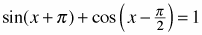3.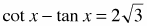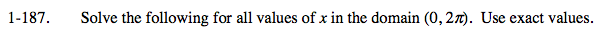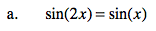sin(2x) = 2sin(x)cos(x). Substitute and solve for x.

There will be four solutions.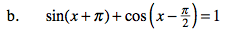$\text{sin}(x+\pi )+\text{cos}\left(x-\frac{\pi}{2}\right)=-\text{sin}(x)+\text{cos}(x)\text{cos}\left(\frac{\pi}{2}\right)+\text{sin}(x)\text{sin}\left ( \frac{\pi}{2} \right )$

There is no solution to this problem.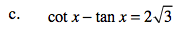$\text{cot}(x)=\frac{\text{cos}(x)}{\text{sin}(x)}\ \ \ \ \ \text{tan}(x)=\frac{\text{sin}(x)}{\text{cos}(x)}$

There will be four solutions.